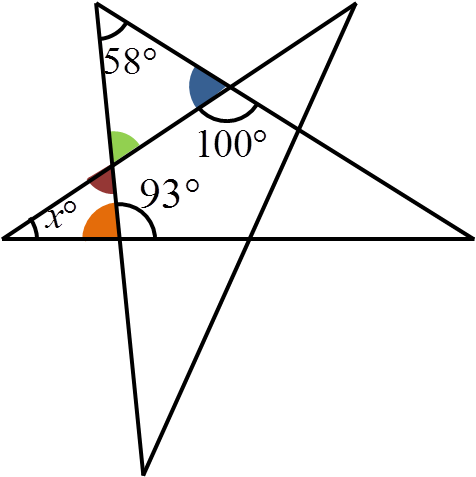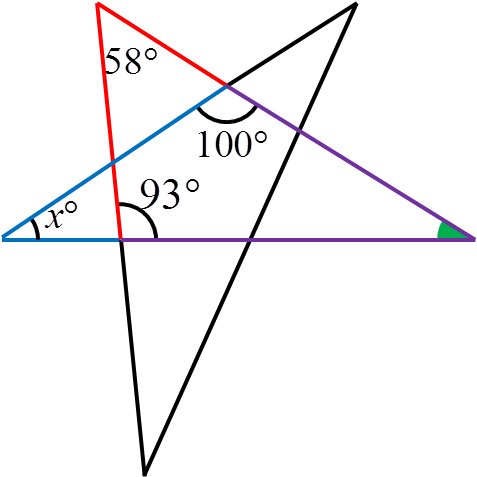#### You may also like### Consecutive Numbers

An investigation involving adding and subtracting sets of consecutive numbers. Lots to find out, lots to explore.### Calendar Capers

Choose any three by three square of dates on a calendar page...### Days and Dates

Investigate how you can work out what day of the week your birthday will be on next year, and the year after...

##### Age 11 to 14 ShortChallenge Level

There are two different ways of calculating the angle $x$.The first method is as follows:

The blue angle can be calculated since angles on a straight line add to $180^\circ$. This means it is $180^\circ - 100^\circ = 80^\circ$.

The orange angle can be calculated similarly, to be $180^\circ - 93^\circ = 87^\circ$.

The green angle can then be calculated since the angles in the top-left triangle add up to $180^\circ$. This is $180^\circ - 80^\circ - 58^\circ = 42^\circ$.

The green and red angles are opposite angles. This means that they are equal, so the red angle is also $42^\circ$.

Then, the angles in the left-hand triangle must add up to $180^\circ$, so $x^\circ = 180^\circ - 42^\circ - 87^\circ= 51^\circ$.The other method involves looking at the farthest right angle in the diagram.

The angles in the red and purple triangle must add up to $180^\circ$, so the green angle is $180^\circ - 58^\circ - 93^\circ = 29^\circ$.

Then, the angles in the blue triangle must also add up to $180^\circ$. Therefore, $x^\circ = 180^\circ - 29^\circ - 100^\circ = 51^\circ$.

This problem is taken from the UKMT Mathematical Challenges.
You can find more short problems, arranged by curriculum topic, in our short problems collection.